CBSE Class 12 Sample Paper for 2018 Boards

Class 12
Solutions of Sample Papers and Past Year Papers - for Class 12 Boards

### Using integration, find the area in the first quadrant bounded by the curve y = x|x|, the circle x 2 + y 2 = 2 and the y-axis

This is a question of CBSE Sample Paper - Class 12 - 2017/18.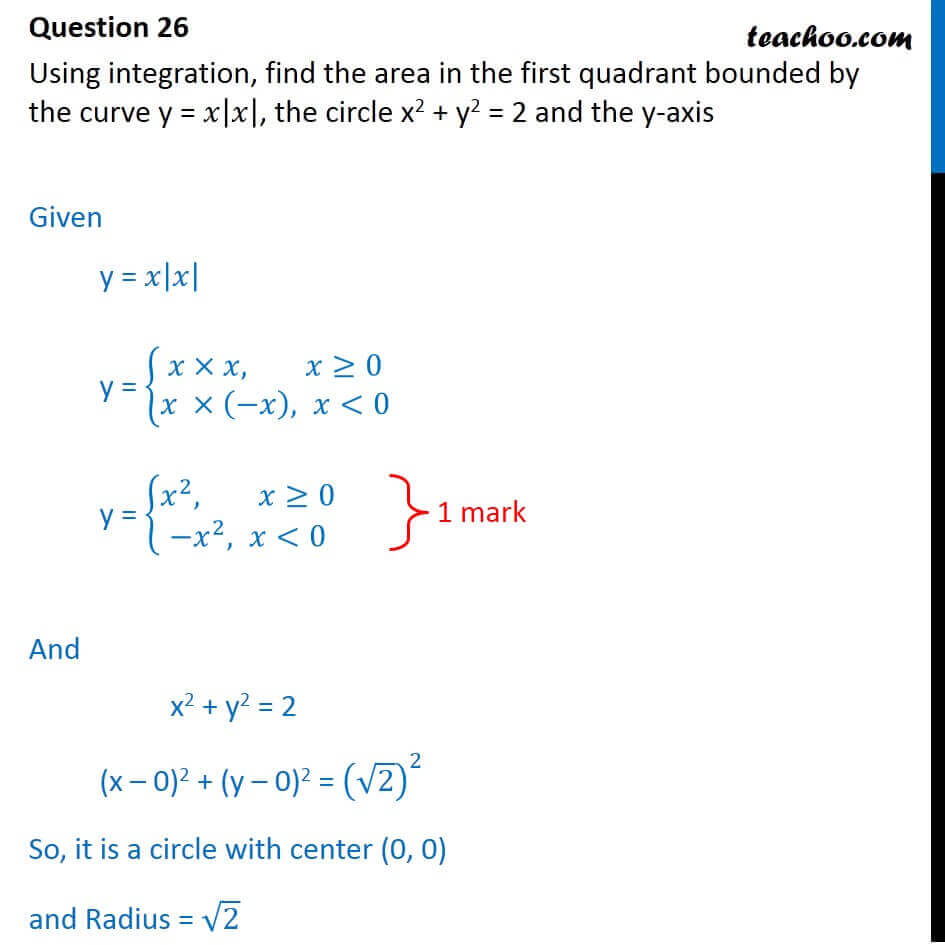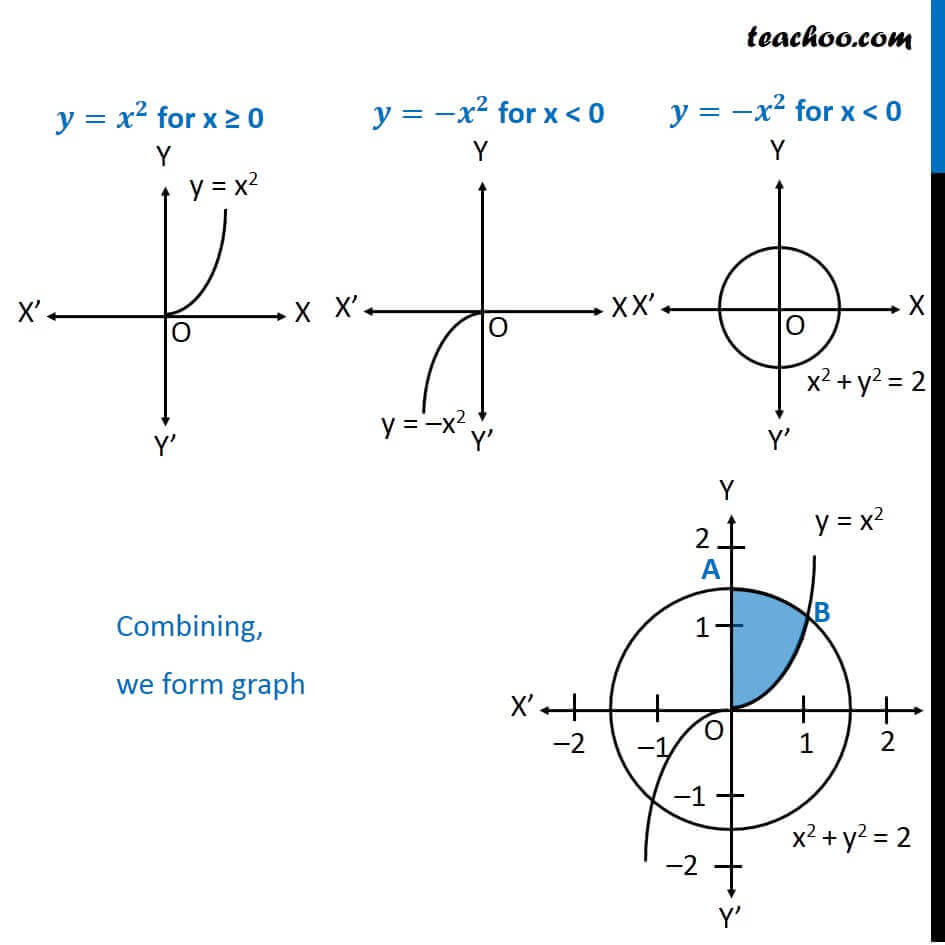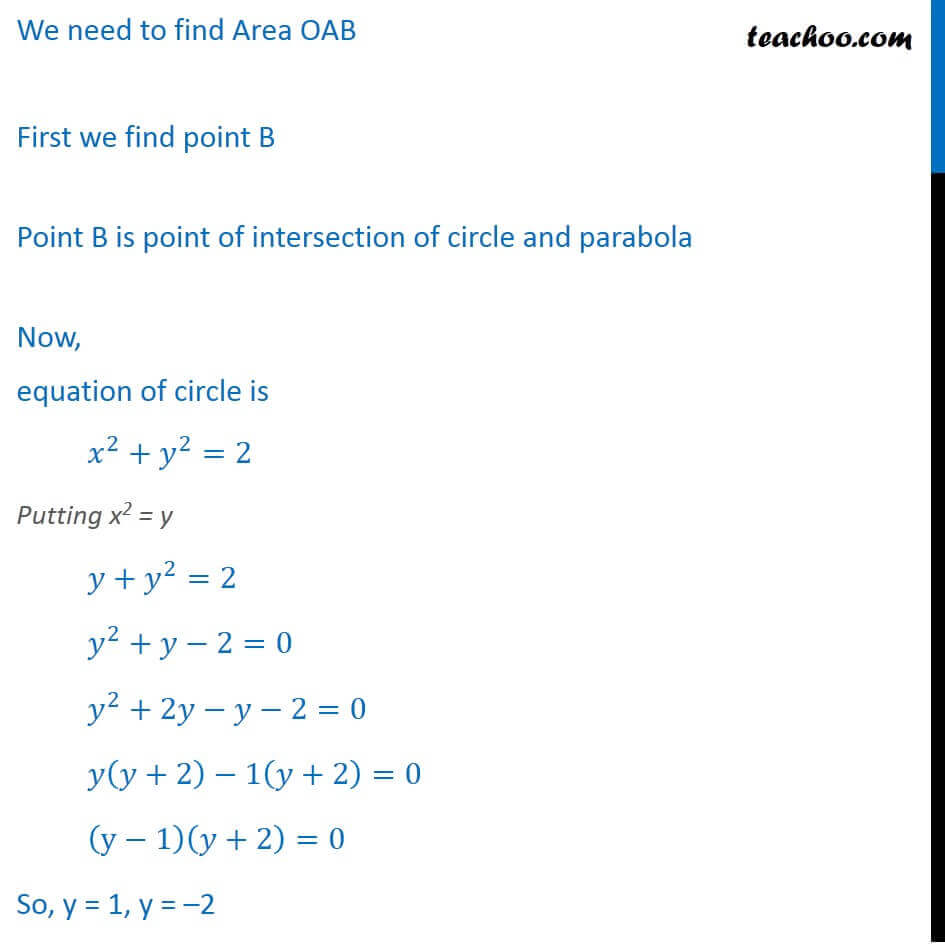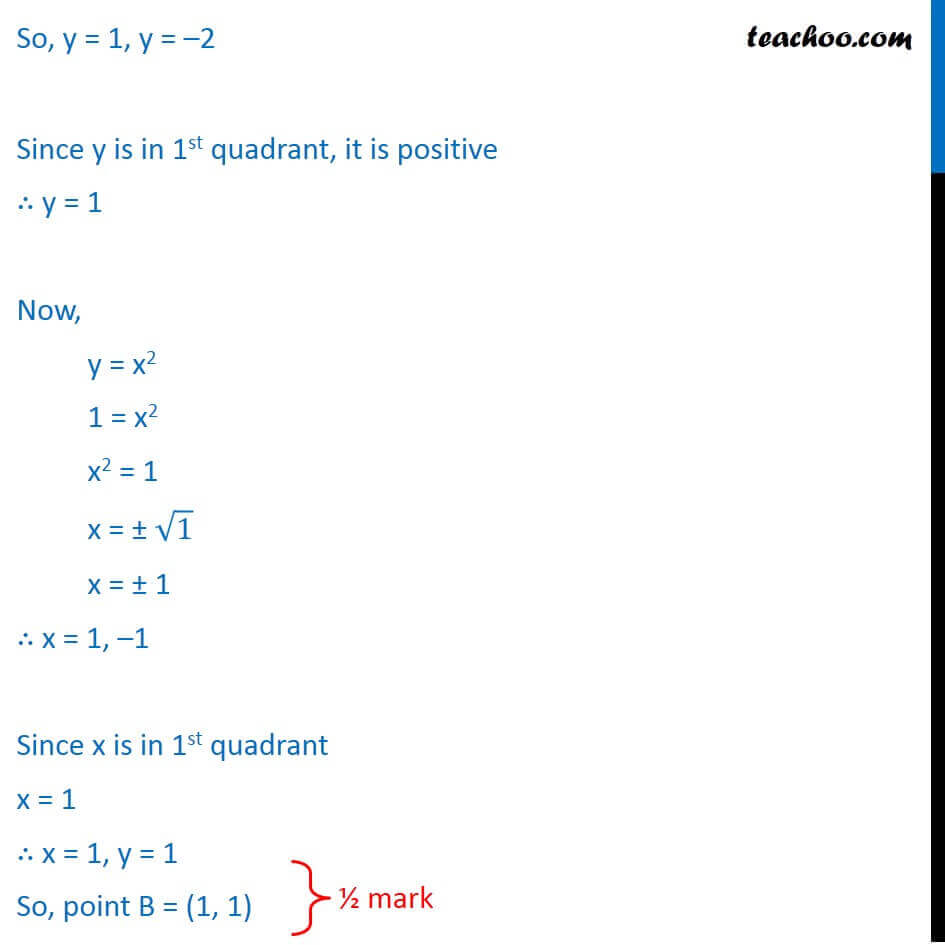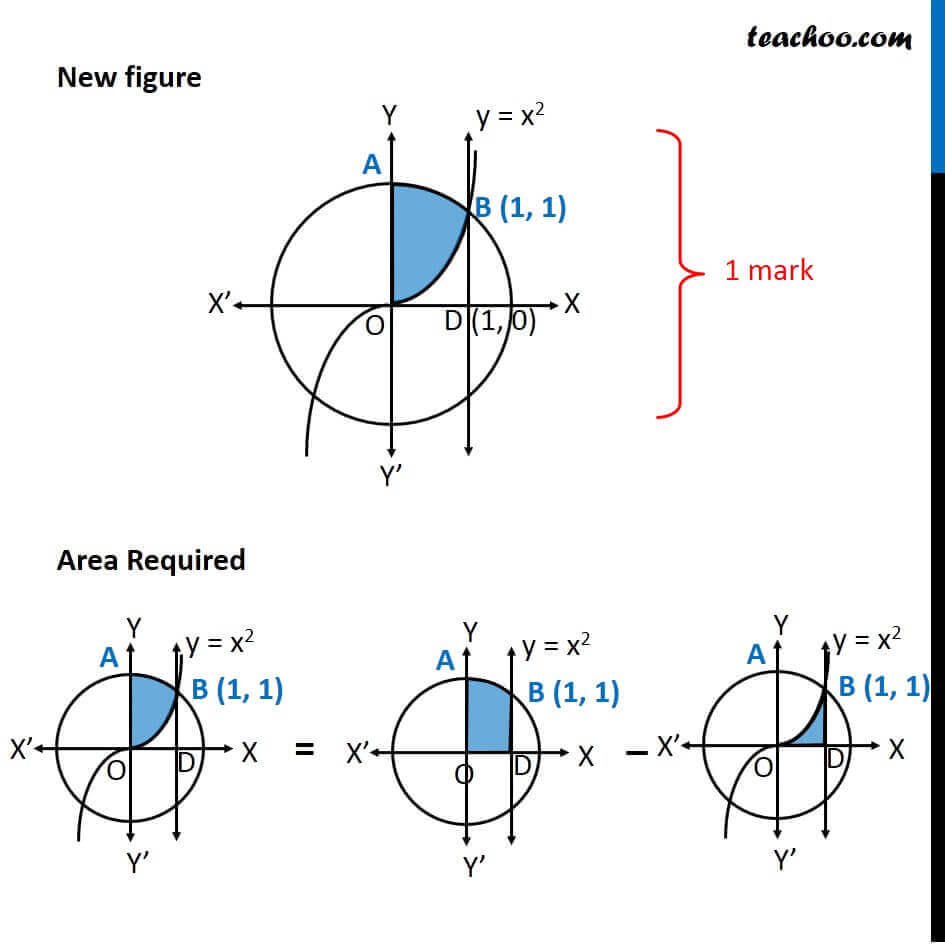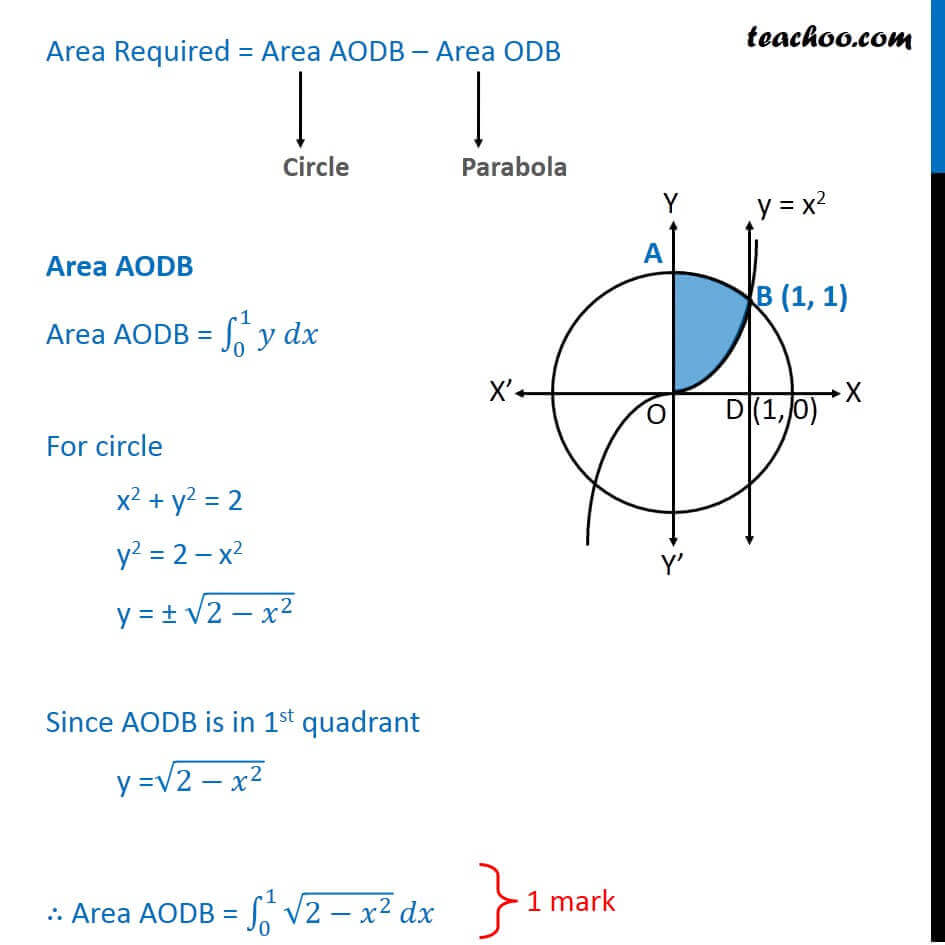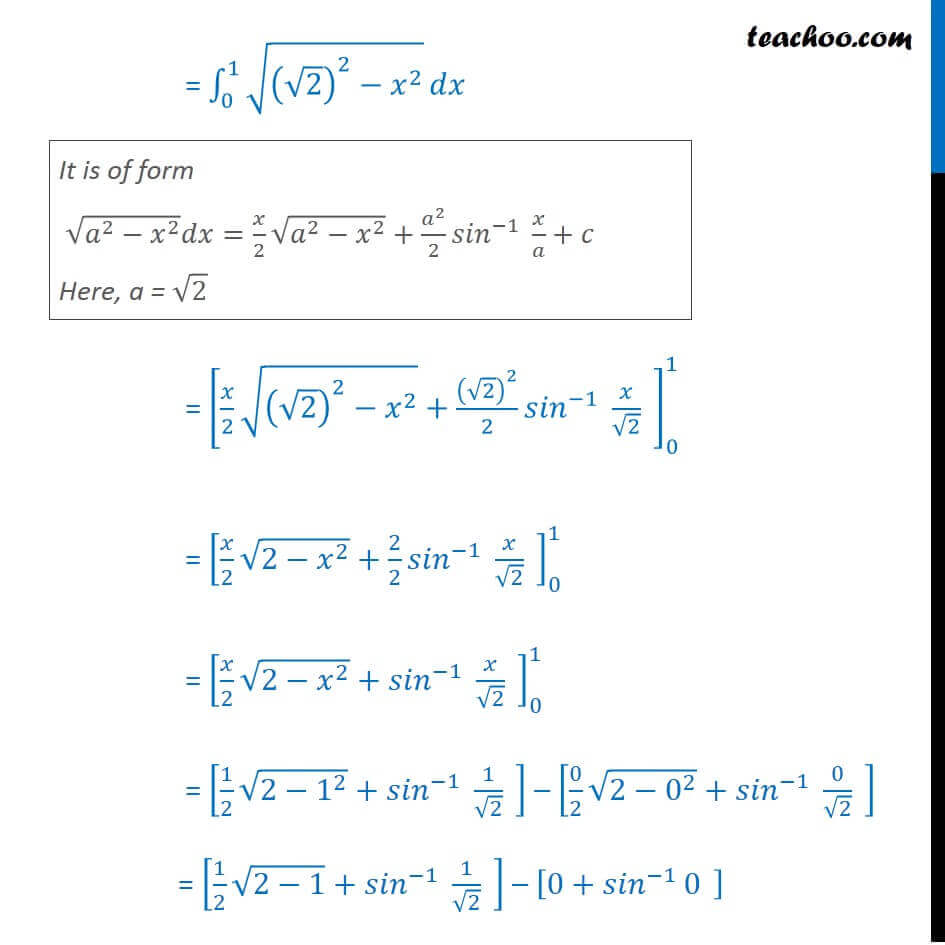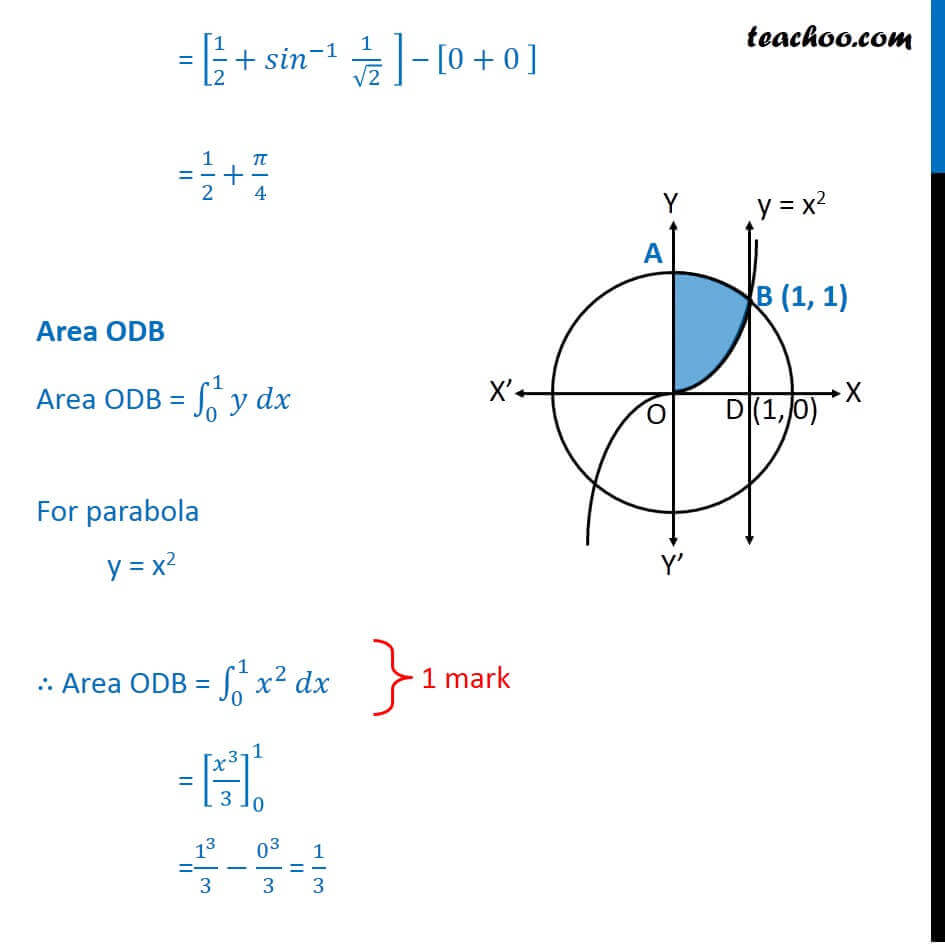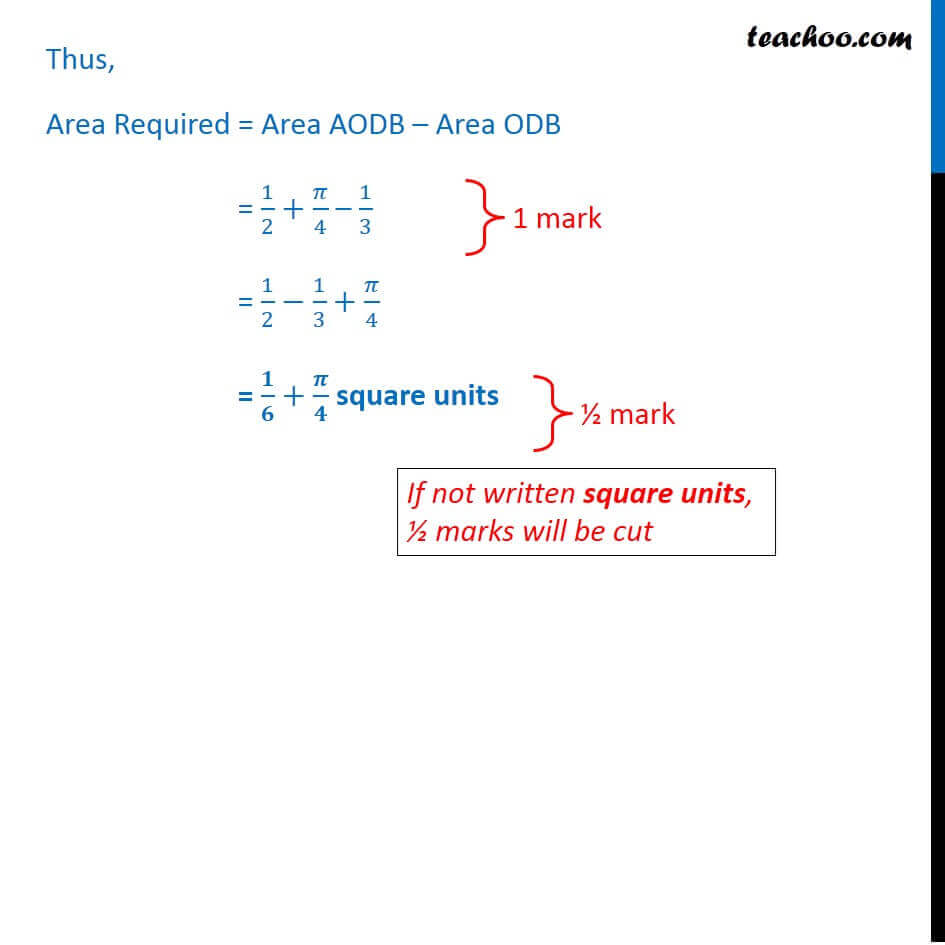Learn in your speed, with individual attention - Teachoo Maths 1-on-1 Class

### Transcript

Question 26 Using integration, find the area in the first quadrant bounded by the curve y = 𝑥|𝑥|, the circle x2 + y2 = 2 and the y-axis Given y = 𝑥|𝑥| y = {█(𝑥×𝑥, 𝑥≥0@𝑥 ×(−𝑥), 𝑥<0)┤ y = {█(𝑥^2, 𝑥≥0@−𝑥^2, 𝑥<0)┤ And x2 + y2 = 2 (x – 0)2 + (y – 0)2 = (√2)^2 So, it is a circle with center (0, 0) and Radius = √2 Question 26 Using integration, find the area in the first quadrant bounded by the curve y = 𝑥|𝑥|, the circle x2 + y2 = 2 and the y-axis Given y = 𝑥|𝑥| y = {█(𝑥×𝑥, 𝑥≥0@𝑥 ×(−𝑥), 𝑥<0)┤ y = {█(𝑥^2, 𝑥≥0@−𝑥^2, 𝑥<0)┤ And x2 + y2 = 2 (x – 0)2 + (y – 0)2 = (√2)^2 So, it is a circle with center (0, 0) and Radius = √2 𝒚=〖−𝒙〗^𝟐 for x < 0 𝒚=〖−𝒙〗^𝟐 for x < 0 Combining, we form graph We need to find Area OAB First we find point B Point B is point of intersection of circle and parabola Now, equation of circle is 𝑥^2+𝑦^2=2 Putting x2 = y 𝑦+𝑦^2=2 𝑦^2+𝑦−2=0 𝑦^2+2𝑦−𝑦−2=0 𝑦(𝑦+2)−1(𝑦+2)=0 (y−1)(𝑦+2)=0 So, y = 1, y = –2 So, y = 1, y = –2 Since y is in 1st quadrant, it is positive ∴ y = 1 Now, y = x2 1 = x2 x2 = 1 x = ± √1 x = ± 1 ∴ x = 1, –1 Since x is in 1st quadrant x = 1 ∴ x = 1, y = 1 So, point B = (1, 1) Area Required = Area AODB – Area ODB Area AODB Area AODB = ∫_0^1▒〖𝑦 𝑑𝑥〗 For circle x2 + y2 = 2 y2 = 2 – x2 y = ± √(2−𝑥^2 ) Since AODB is in 1st quadrant y =√(2−𝑥^2 ) ∴ Area AODB = ∫_0^1▒√(2−𝑥^2 ) 𝑑𝑥 = ∫_0^1▒√((√2)^2−𝑥^2 ) 𝑑𝑥 It is of form √(𝑎^2−𝑥^2 ) 𝑑𝑥=𝑥/2 √(𝑎^2−𝑥^2 )+𝑎^2/2 〖𝑠𝑖𝑛〗^(−1)⁡〖 𝑥/𝑎+𝑐〗 Here, a = √2 = [𝑥/2 √((√2)^2−𝑥^2 )+(√2)^2/2 〖𝑠𝑖𝑛〗^(−1)⁡〖 𝑥/√2〗 " " ]_0^1 = [𝑥/2 √(2−𝑥^2 )+2/2 〖𝑠𝑖𝑛〗^(−1)⁡〖 𝑥/√2〗 " " ]_0^1 = [𝑥/2 √(2−𝑥^2 )+〖𝑠𝑖𝑛〗^(−1)⁡〖 𝑥/√2〗 " " ]_0^1 = [1/2 √(2−1^2 )+〖𝑠𝑖𝑛〗^(−1)⁡〖 1/√2〗 " " ] – [0/2 √(2−0^2 )+〖𝑠𝑖𝑛〗^(−1)⁡〖 0/√2〗 " " ] = [1/2 √(2−1)+〖𝑠𝑖𝑛〗^(−1)⁡〖 1/√2〗 " " ] – [0+〖𝑠𝑖𝑛〗^(−1)⁡0 " " ] = [1/2+〖𝑠𝑖𝑛〗^(−1)⁡〖 1/√2〗 " " ] – [0+0" " ] = 1/2+𝜋/4 Area ODB Area ODB = ∫_0^1▒〖𝑦 𝑑𝑥〗 For parabola y = x2 ∴ Area ODB = ∫_0^1▒𝑥^2 𝑑𝑥 = [𝑥^3/3]_0^1 =1^3/3−0^3/3 = 1/3 Thus, Area Required = Area AODB – Area ODB = 1/2+𝜋/4 – 1/3 = 1/2−1/3+𝜋/4 = 𝟏/𝟔+𝝅/𝟒 square units